## Journal of Physics Research and Applications

All submissions of the EM system will be redirected to Online Manuscript Submission System. Authors are requested to submit articles directly to Online Manuscript Submission System of respective journal.

Research Article,  J Phys Res Appl Vol: 1 Issue: 1

# The Effect of Drifting Lighter Ions on Solitary Waves in Heavier, Pair Ion Plasmas with Kappa Described Electrons

Varghese A, Willington NT, Hussein T, Shilpa S and Venugopal C*

School of Pure and Applied Physics, Mahatma Gandhi University, Kottayam, Kerala, India

*Corresponding Author : Chandu Venugopal
School of Pure and Applied Physics, Mahatma Gandhi University, Kottayam, Kerala, India
Tel: (+91) 9446196143
E-mail: cvgmgphys@yahoo.co.in

Received: November 08, 2017 Accepted: December 20, 2017 Published: December 29, 2017

Citation: Varghese A, Willington NT, Hussein T, Shilpa S, Venugopal C (2017) The Effect of Drifting Lighter Ions on Solitary Waves in Heavier, Pair Ion Plasmas with Kappa Described Electrons. J Phys Res Appl 1:1.

## Abstract

We investigate the effect of drifting ions on compressive and rarefactive solitary waves in a five component plasma. Heavier pair ions, two components of electrons and drifting lighter ions form the five components. We use the pseudo-potential method to investigate the conditions for the existence of solitary waves. We find that both the Sagdeev potential and amplitudes of solitary waves are dependent on the velocities of the drifting ions: the amplitude of both compressive and rarefactive solitary waves increases with increasing drifting velocities of the lighter ions.

## Introduction

A conventional electron-ion plasma supports a wide variety of nonlinear waves. These waves have been thoroughly researched since the path breaking study of Washimi and Taniuti , who investigated small amplitude ion acoustic (IA) waves in an unmagnetized plasma consisting of cold ions and hot, isothermal electrons by deriving the well known K-dV equation. At about the same time, Sagdeev  used his pseudo-potential approach to study the basic properties of arbitrary amplitude IA solitary waves. This study was quickly extended to plasmas containing two components of electrons with different temperatures [3,4]. More than one component of electrons, gave way to more than one component of ions and Ferrante  studied the properties of IA solitons in two and three ion plasmas.

Owing to the ease with which it could be observed, the pioneering studies mentioned above were extended in every possible way. Thus various aspects of IA solitary waves were again explored in two temperature electron plasmas [6-13], multi-ion plasmas [14-21], non- Maxwellian component plasmas [22-32], finite ion temperature plasmas [33,34], pair (electron-positron)-ion plasmas [35,36] and heavier pair ion with another lighter ion component plasmas [37-40]. In addition to the very general plasma compositions and models mentioned above, the effect of streaming plasma components on ion acoustic and dust acoustic solitary waves has also been considered. The effect of streaming ions [41-46], streaming electrons [44,47,48] and even streaming dust [49,50,51] has also been exhaustively investigated.

cometary plasma is a genuine multi-ion plasma. While positively charged oxygen ions were expected to be a component formed by the dissociation of water molecules , other positively charged ions such as H2 +, He+, He2 +, C+, OH+, H2O+, H30+, CO+, S+ etc. have also been observed [53,54]. However, it was the unexpected discovery of negative ions, in three broad mass peaks of 7-19, 22-65 and 85-110 amu with energies ranging from .03 to 3.0 keV, that made cometary plasmas genuine multi ion plasmas . And, the unambiguous identification of negatively charged oxygen ions (Oˉ), opened up the exciting prospect of modelling O+ and Oˉ as an ion pair [37,38,40,56].

As regards electrons, observations by the spacecraft ICE at comet Giacobini-Ziner revealed the existence of three components of electrons: a cold, mid, and hot component where the mid component was the photo-electrons generated by the photo-ionization of water molecules . In a related modelling study , analysis of gas production rates at comets, noticed a double peak structure of photo-electrons. And in a very recent modelling study, two kappa distributions were fitted to the observations of electrons made at comet 67P/Churyumov-Gerasimenko .

Thus our plasma is composed of five components: two components of electrons, both described by kappa distributions, drifting hydrogen ions (to model the solar wind impacting the comet) and positively and negatively charged oxygen ions. Previous studies on the influences of streaming components have been mentioned above.

We find that the plasma supports both rarefactive and compressive solitons . Also the amplitudes of both types of solitons are sensitively dependent on the drift velocity of the lighter ions.

Basic equations

As mentioned above we are interested in studying the effect of streaming ions on IA solitary waves in a five component plasma . The five components are a pair of oppositely charged heavier ions, a streaming lighter ion component and two components of electrons  (a hotter solar component denoted by ‘se’ and a colder cometary component of photoelectrons denoted by ‘ce’). The dimensionless distribution of the electron components is given by(1)

In (1) nj is the dimensionless density normalized by its equilibrium value. ѱ is the potential normalized bywhere ‘e’ is the electronic charge and kB is the Boltzmann’s constant. T1 is the temperature of the negatively charged heavier ions.is the dimensionless temperature and κj are the spectral indices .

In what follows, ‘i’ denotes streaming ions while the indices 1 and 2 would respectively denote negatively and positively charged heavier ions . Thus the equations of motion for the drifting ions and the heavier ions are given by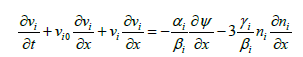(2)(3)(4)

In (2) to (4), ‘x’ and ‘t’ have been normalized respectively by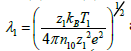and. All velocities are normalized by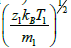with νi0 now being the normalized drift velocity of drifting ions. Also these adiabatically heated ions obey(5)

In the above, symbols n denote the densities; z, the charge numbers and m, the masses. The normalized equation of continuity for the streaming ion is(6)

While that for the heavier ions is(7)

Finally, we need the Poisson’s equation, which in its dimensionless form, is given by(8)

In (8)(9)

where the index ‘0’ indicates equilibrium values of densities.

Nonlinear analysis

We now use equations (2) to (8) for a nonlinear analysis. We therefore define a transformed coordinate ξ = x-Mt where M is the speed of the localized structure moving with the frame . Assuming that all dependent variables are functions of ξ, we can, from (6), obtain the expression for the streaming ion density as(10)

Expressions for n1 and n2 can be easily got from (10) by putting νi0 = 0 and replacing νi respectively by ν1 or ν2. Similarly, from (2) we obtain(11)

Where,(12)It may be noted at this point that ni reduces to the corresponding expression in Shan and Mushtaq  for αi = βi = γi =1. Similar expressions can be obtained for both ν1 and ν2.

Having thus obtained expressions for ni, n1 and n2 and for nse and nce from (1) and substituting into (8) and following standard procedures allows one to write down the energy integral as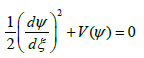(13)

Where V(ѱ) is now given by(14)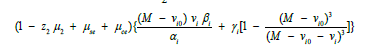where(15)(16)

As a check on (14) we note that it resembles the expression for the Sagdeev potential in  if allowance is made for the extra components in our model. From the condition for the existence of solutions [37,66], we can show that the critical value of M for the existence of the two types of solitons (rarefactive and compressive) is(17)

whereandThe small amplitude approximation for V(ѱ) is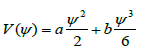(18)

where(19)

and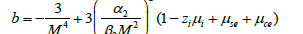(20)As a check on our results we find that expressions (18) and (19) closely resemble that given in  if allowance is made for the fact that we now have drifting ions.

The soliton solutions for potentials of the type (14) is given by  aswhereis the amplitude andis the width of the soliton.

#### Results

Though our relations are applicable to any plasma, we are interested here in parameters relevant to comet Halley. The density of hydrogen ions observed at the comet was ni0 = 4.95 cm-3 with a temperature Ti=8×104 K. The solar electron temperature at the comet was Tse=2×105 K . Hence the temperature of the colder photoelectrons was set at Tce=2×104 K. The Oˉ ions were observed with an energy ~1 eV with densities ≤ 1cm-3 . Thus we keep n10=0.05 cm-3 and n20 = 0.5 cm-3.

Figure 1 depicts the Sagdeev potential V (ѱ) and soliton structure for the first solution for densities ni0 = 4.95 cm-3, n20 = 0.5 cm-3 and n10 = 0.05 cm-3, temperatures Tse=2×105 K, Tce =1.5×104 K and Ti = 8×104 K, T1=T2=2.8×104 K, charge numbers z1 =5, z2 =3 and zi =1, M = 1.2, spectral indices κse = 3 and κce = 5 as a function of drift velocities νi0 = 0 ( curve (a)), νi0 = 0.4 ( curve (b)) and νi0 = 1.0 ( curve (c)). The figure shows that the depth of the potential V (ѱ) increases with increasing νi0. Also the magnitude of the amplitude of the solitary wave, which is rarefactive in nature, also increases with increasing νi0.

Figure 1: Variation of Sagdeev potential V (ψ ) and solitary wave amplitude with drift velocityFigure 2 also depicts the Sagdeev potential V(ѱ) and solitary structure for the second solution for densities ni0 = 4.95 cm-3, n20 = 0.5 cm-3 and n10 = 0.05 cm-3, temperatures Tse=2×105 K, Tce =1.5×104 K and Ti = 8×104 K, T1=T2=2.8×104 K, charge numbers z1 =5, z2 =3 and zi =1, M = 1.2, spectral indices κse = 3 and κce = 5 as a function of drift velocities νi0 = 0 ( curve (a)), νi0 = 0.4 ( curve (b)) and νi0 = 1.0 ( curve (c)). The figure shows that the depth of the potential V(ѱ) increases with increasing νi0. Also the magnitude of the amplitude of the solitary wave, which is now compressive in nature, also increases with increasing νi0.

Figure 2: Variation of Sagdeev potential V (ψ ) and solitary wave amplitude with drift velocitySimilar to Figure 1, the amplitude of the rarefactive solitons increases with increasing ni0, Ti and T1 and decreases with increasing κse, z1, z2 and n20. This is true for both νi0 = 1.0 and νi0 ≠ 0 .

And similar to Figure 2, the amplitude of the compressive solitons increases with increasing ni0, Ti and T1 and decreases with increasing κse and n20. This is true for both νi0 = 1.0 and νi0 ≠ 0 .

Also a comparison of Figures 1 and 2 show that the amplitude of rarefactive solitons is of much greater magnitude than the compressive solitons.

## Conclusions

We have investigated the effect of drifting lighter ions on rarefactive and compressive solitary waves in a five component cometary plasma. Drifting lighter ions, heavier pair ions and two components of electrons, described by kappa distributions, form the five components. We find that the amplitude of the solitary waves is sensitively dependent on the drift velocities of the lighter ions: the amplitudes increase with increasing drift velocities, increasing lighter ion densities and temperatures of these ions. Thus these solitary waves are most likely to be observed under disturbed solar conditions when these parameters of the solar wind are large. And finally our relations can also be considered as generalizations of earlier studies [37,66].

## References## Explore SciTechnol

###### Citations : 19

Journal of Physics Research and Applications received 19 citations as per Google Scholar report

## Recommended Conferences

### 9th International Conference on Physics

Edinburgh, Scotland

### 6th International Conference on Astronomy, Astrophysics and Space Science

Budapest, Hungary